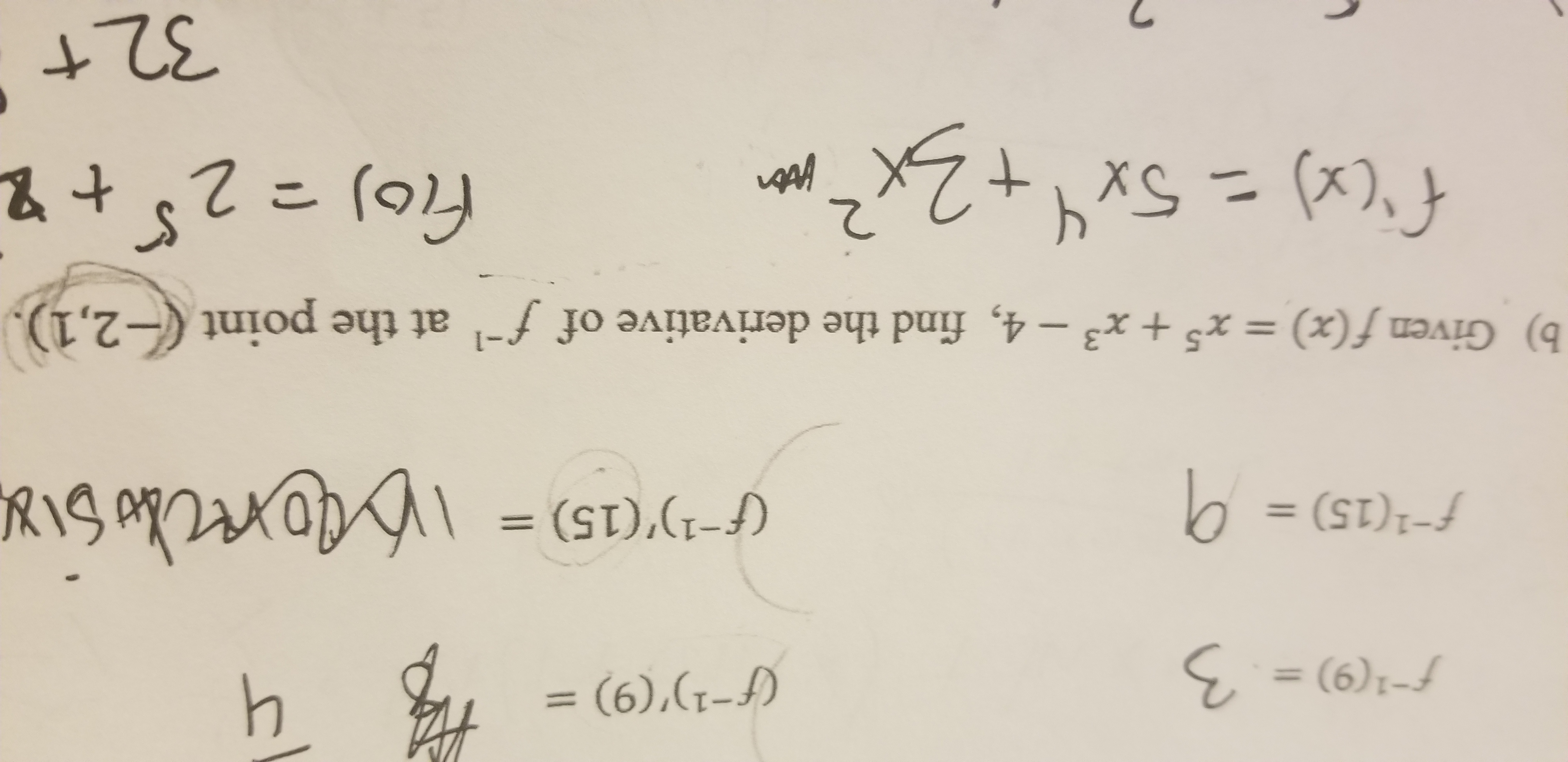# 41 {(9) = . 3 f1'(9) f(15) = 0 (f1)'(15) = b) Given f(x) = x5 + x3 - 4, find the derivative of f -2,1). at the point fio) 2 t2 32 t

Question

Given f(x) = x^5 + x^3 - 4 , find the derivative of f inverse at the point (-2,1).help_outlineImage Transcriptionclose41 {(9) = . 3 f1'(9) f(15) = 0 (f1)'(15) = b) Given f(x) = x5 + x3 - 4, find the derivative of f -2,1). at the point fio) 2 t2 32 t fullscreen

## Expert Answer

### Want to see this answer and more?

Experts are waiting 24/7 to provide step-by-step solutions in as fast as 30 minutes!*

*Response times vary by subject and question complexity. Median response time is 34 minutes and may be longer for new subjects.
Tagged in
MathCalculus

### Derivative© 2021 bartleby. All Rights Reserved.